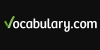### Calculate• (v. i.) To ascertain or determine by mathematical processes, usually by the ordinary rules of arithmetic; to reckon up; to estimate; to compute. • (v. i.) To ascertain or predict by mathematical or astrological computations the time, circumstances, or other conditions of; to forecast or compute the character or consequences of; as, to c...
Found on http://thinkexist.com/dictionary/meaning/calculate/

### calculateverb judge to be probable
Found on https://www.encyclo.co.uk/local/20974

### calculatecipher verb make a mathematical calculation or computation
Found on https://www.encyclo.co.uk/local/20974

### CalculateCal'cu·late intransitive verb [ imperfect & past participle Calculater ; present participle & verbal noun Calculating .] [ L, calculatus , past participle of calculate , from calculus a pebble, a stone used in recko...
Found on http://www.encyclo.co.uk/webster/C/7

### calculatemake a mathematical computation
Found on https://www.vocabulary.com/lists/464499

### calculate[v] - specifically design a product, event, or activity for a certain public 2. [v] - judge to be probable 3. [v] - make a mathematical calculation or computation
Found on http://www.webdictionary.co.uk/definition.php?query=calculate
No exact match found